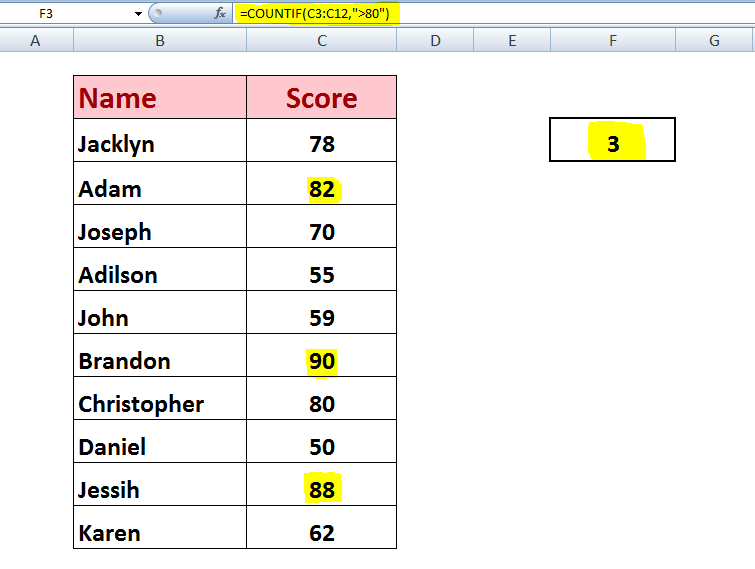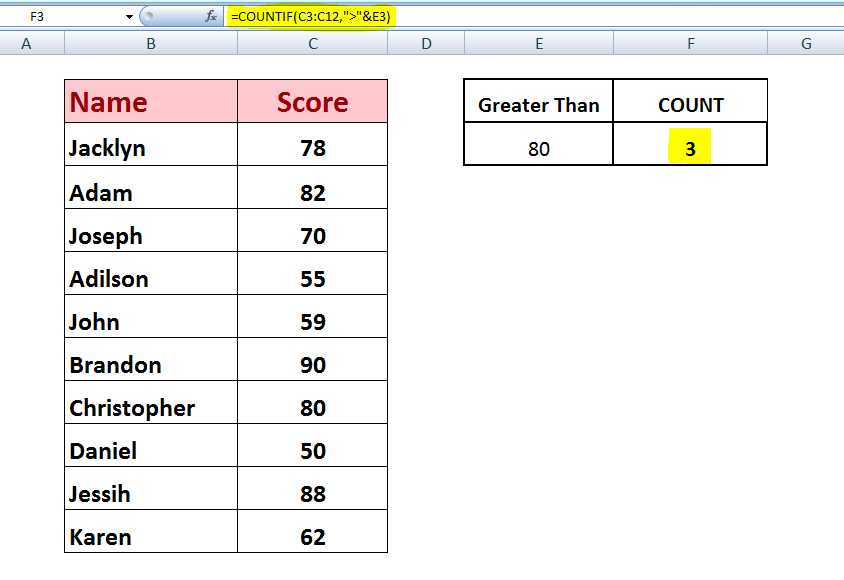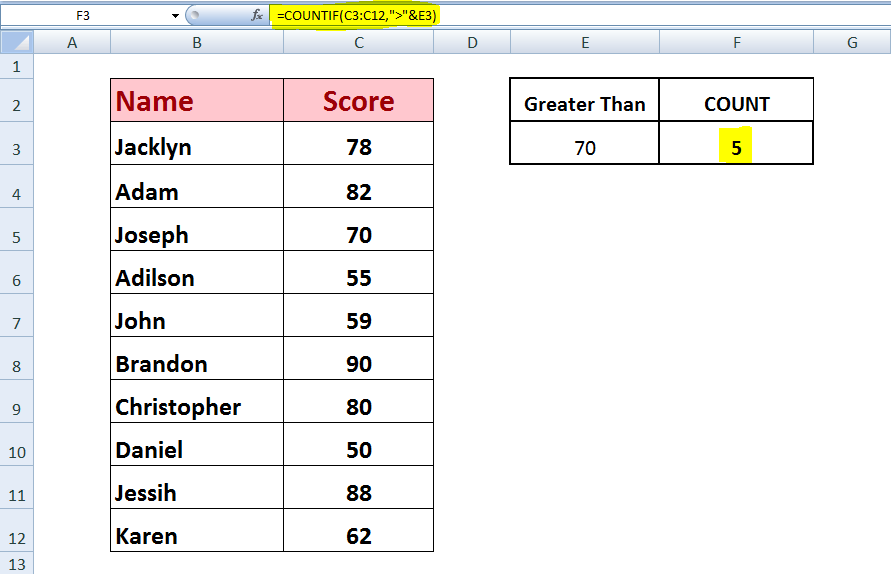Get instant live expert help with Excel or Google Sheets“My Excelchat expert helped me in less than 20 minutes, saving me what would have been 5 hours of work!”

#### Post your problem and you’ll get expert help in seconds.

Your message must be at least 40 characters
Our professional experts are available now. Your privacy is guaranteed.

# Learn How to Use COUNTIF to Count Cells Greater Than Some Number

Do you have a group of data in Excel and need to find the number of cells that are greater than a particular number? If so, you can use the COUNTIF function for this purpose.

### Use COUNTIF to figure cells greater than some chosen figure

The COUNTIF function counts the number of cells that meet specified criteria. It can count the number of cells that contain a numeric value greater than a specified number as criteria.
The syntax of COUNTIF contains a range of data cells that you want to test against a criterion value with comparison operator of greater than (>)The syntax for the COUNTIF function is:

`=COUNTIF (range, criterion)`

Comparison operators > (greater than) is used in the expression to check the criterion with a numeric value. Whole criterion expression must be enclosed in double quotation marks, such as “>80.”

So the active cell will contain formula like;

`=COUNTIF (A1:A20, “>80”)`

But if criteria value is provided in a cell reference, then criterion expression can consist of a comparison operator and cell reference. In this case, the comparison operator and cell reference are joined together by using an ampersand (&), which is a concatenating operator, such as “>”&C2

Here, comparison operator must be enclosed in double quotation marks, while cell reference should not be enclosed in double quotation marks.

So the active cell will contain formula like;

`=COUNTIF (A1:A20, “>”&C2)`

Now COUNTIF function will count the number of cells in the selected data range that contain a numeric value greater than the specified numeric value in criterion expression and will return the result as a number.

In this article, we have a student scorecard and want to count the number of students that have a score greater than a specific score as criteria. Suppose we want to count the number of students who have scored greater than 80 marks, and we use COUNTIF function in active cell F3 to show the results as a number, like;

`=COUNTIF(C3:C12,">80")`

Here, cell range C3:C12 contains the scoring marks of all the students, and we want to test these scores against criteria, greater than 80 marks, in double quotation marks in criterion expression.

COUNTIF function counts the number of cells that contain a value greater than 80 and return the output as number as shown below.Here, you can see COUNTIF has returned the results as a number, representing only three students who have scored more than 80 marks, as highlighted above.

We can also mention our criteria value in a cell reference and we can put this cell reference in criterion expression along with comparison operator, greater than (>), joined by ampersand operator (&).

We have placed our criteria value in cell reference E3, and we have placed COUNTIF function in active cell F3 to show the result.

`=COUNTIF(C3:C12,">"&E3)`

You can see comparison operator is enclosed in double quotation marks, and cell reference is without double quotation marks, both joined by an ampersand (&), in criterion expression of COUNTIF function.Both methods are easy, but the cell reference approach is handier because you can easily modify your criteria value in cell reference without editing your COUNTIF function. It will update the results based on the criteria entered in the cell reference.

Like, if you want to count the students who have scored more than 70 marks instead of 80, then you can easily insert this new criterion in cell reference E3 and COUNTIF function will update the results accordingly.Still need some help with Excel formatting or have other questions about Excel? Connect with a live Excel expert here for some 1 on 1 help. Your first session is always free.

### Did this post not answer your question? Get a solution from connecting with the expert.Another blog reader asked this question today on Excelchat:
Related blogs
Solution examplesI need a formula to count the number of rows that contain at least one of two possible phrases, but not give me the total number of instances that both phrases occur overall.
Solved by E. B. in 33 minsi have a table with Dates, First names , Last names and i want to count how many times a name occurs, but if a name occurs more than once on a particular date i need to only count it once. this is on excel and not google sheets
Solved by F. H. in 31 minsNeed a formula that counts (1,2,3,4 ect..in cell M21) a range of cells, N4:N20, which are annual premium values. But I only want to add a sale count if the average monthly premium is above \$30 per sale. So if someone has 4 sales but they don't equal a total of \$120/mth (\$30 x 4 = \$120)... they would only get a count of 3 sales.
Solved by S. E. in 60 minsHello, I am trying to create a total color column? Can anyone help?
Solved by I. A. in 60 minsAny rep that has orders over 500 and occurrences at zero for each month, for a 6 month period. I need a sum
Solved by C. E. in 60 mins## Subscribe to Excelchat.coAnother blog reader asked this question today on Excelchat: